# dsphdl.IFFT

Compute inverse fast Fourier transform (IFFT)

## Description

The `dsphdl.IFFT` System object™ provides two architectures to optimize either throughput or area. Use the streaming Radix 2^2 architecture for high-throughput applications. This architecture supports scalar or vector input data. You can achieve gigasamples-per-second (GSPS) throughput using vector input. Use the burst Radix 2 architecture for a minimum resource implementation, especially with large FFT sizes. Your system must be able to tolerate bursty data and higher latency. This architecture supports only scalar input data. The object accepts real or complex data, provides hardware-friendly control signals, and has optional output frame control signals.

To calculate the inverse fast Fourier transform:

1. Create the `dsphdl.IFFT` object and set its properties.

2. Call the object with arguments, as if it were a function.

## Creation

### Syntax

``IFFT_N = dsphdl.IFFT``
``IFFT_N = dsphdl.IFFT(Name,Value)``

### Description

````IFFT_N = dsphdl.IFFT` returns an HDL IFFT System object, `IFFT_N`, that performs a fast Fourier transform. ```

example

````IFFT_N = dsphdl.IFFT(Name,Value)` sets properties using one or more name-value pairs. Enclose each property name in single quotes.Example: `ifft128 = dsphdl.IFFT('FFTLength',128)````

## Properties

expand all

Unless otherwise indicated, properties are nontunable, which means you cannot change their values after calling the object. Objects lock when you call them, and the `release` function unlocks them.

If a property is tunable, you can change its value at any time.

Hardware implementation, specified as either:

• `'Streaming Radix 2^2'` — Low-latency architecture. Supports gigasamples-per-second (GSPS) throughput when you use vector input.

• `'Burst Radix 2'`— Minimum resource architecture. Vector input is not supported when you select this architecture. When you use this architecture, your input data must comply with the `ready` backpressure signal.

HDL implementation of complex multipliers, specified as either ```'Use 4 multipliers and 2 adders'``` or ```'Use 3 multipliers and 5 adders'```. Depending on your synthesis tool and target device, one option may be faster or smaller.

Order of the output data, specified as either:

• `true` — The output channel elements are bit reversed relative to the input order.

• `false` — The output channel elements are in linear order.

The IFFT algorithm calculates output in the reverse order to the input. When you request output in the same order as the input, the algorithm performs an extra reversal operation. For more information on ordering of the output, see Linear and Bit-Reversed Output Order.

Expected order of the input data, specified as either:

• `true` — The input channel elements are in bit-reversed order.

• `false` — The input channel elements are in linear order.

The IFFT algorithm calculates output in the reverse order to the input. When you request output in the same order as the input, the algorithm performs an extra reversal operation. For more information on ordering of the output, see Linear and Bit-Reversed Output Order.

Output scaling, specified as either:

• `true` — The object implements an overall 1/N scale factor by dividing the output of each butterfly multiplication by 2. This adjustment keeps the output of the IFFT in the same amplitude range as its input.

• `false` — The object avoids overflow by increasing the word length by one bit after each butterfly multiplication. The bit growth is the same for both architectures.

Number of data points used for one FFT calculation, specified as an integer power of 2 between 22 and 216. The object accepts FFT lengths outside this range, but they are not supported for HDL code generation.

Enable `reset` input argument to the object. When `reset` is `1` (true), the object stops the current calculation and clears internal states. When the `reset` is `0` (false) and the input `valid` is `1` (true), the object captures data for processing.

Enable `startOut` output argument of the object. When enabled, the object returns an additional output signal that is `true` on the first cycle of each valid output frame.

Enable `endOut` output argument of the object. When enabled, the object returns an additional output signal that is `true` on the first cycle of each valid output frame.

Rounding mode used for fixed-point operations. When the input is any integer or fixed-point data type, the IFFT algorithm uses fixed-point arithmetic for internal calculations. This option does not apply when the input is single or double type. Rounding applies to twiddle factor multiplication and scaling operations.

## Usage

### Syntax

``````[Y,validOut] = IFFT_N(X,validIn)``````
``````[Y,validOut,ready] = IFFT_N(X,validIn)``````
``````[Y,startOut,endOut,validOut] = IFFT_N(X,validIn)``````
``````[Y,validOut] = IFFT_N(X,validIn,resetIn)``````
``````[Y,startOut,endOut,validOut] = IFFT_N(X,validIn,resetIn)``````

### Description

example

``````[Y,validOut] = IFFT_N(X,validIn)``` returns the inverse fast Fourier transform (IFFT), `Y`, of the input, `X`, when `validIn` is `true`. `validIn` and `validOut` are logical scalars that indicate the validity of the input and output signals, respectively.```
``````[Y,validOut,ready] = IFFT_N(X,validIn)``` returns the inverse fast Fourier transform (IFFT) when using the burst Radix 2 architecture. The `ready` signal indicates when the object has memory available to accept new input samples. You must apply input `data` and `valid` signals only when `ready` is `1` (true). The object ignores the input `data` and `valid` signals when `ready` is `0` (false).To use this syntax, set the `Architecture` property to `'Burst Radix 2'`. For example: IFFT_N = dsphdl.IFFT(___,'Architecture','Burst Radix 2'); ... [y,validOut,ready] = IFFT_N(x,validIn)```
``````[Y,startOut,endOut,validOut] = IFFT_N(X,validIn)``` also returns frame control signals `startOut` and `endOut`. `startOut` is `true` on the first sample of a frame of output data. `endOut` is `true` for the last sample of a frame of output data. To use this syntax, set the `StartOutputPort` and `EndOutputPort` properties to `true`. For example: IFFT_N = dsphdl.IFFT(___,'StartOutputPort',true,'EndOutputPort',true); ... [y,startOut,endOut,validOut] = IFFT_N(x,validIn)```
``````[Y,validOut] = IFFT_N(X,validIn,resetIn)``` returns the IFFT, `Y`, when `validIn` is `true` and `resetIn` is `false`. When `resetIn` is `true`, the object stops the current calculation and clears all internal state. To use this syntax, set the `ResetInputPort` property to `true`. For example: IFFT_N = dsphdl.IFFT(___,'ResetInputPort',true); ... [y,validOut] = IFFT_N(x,validIn,resetIn)```
``````[Y,startOut,endOut,validOut] = IFFT_N(X,validIn,resetIn)``` returns the IFFT, `Y`, using all optional control signals. You can use any combination of the optional port syntaxes.```

### Input Arguments

expand all

Input data, specified as a scalar or column vector of real or complex values, in fixed-point or integer format. Vector input is supported with ```'Streaming Radix 2^2'``` architecture only. The vector size must be a power of 2 between 1 and 64 that is not greater than the FFT length.

`double` and `single` data types are supported for simulation, but not for HDL code generation.

Data Types: `fi` | `int8` | `int16` | `int32` | `int64` | `uint8` | `uint16` | `uint32` | `single` | `double`
Complex Number Support: Yes

Control signal that indicates if the input data is valid. When `validIn` is `1` (`true`), the object captures the values from the `dataIn` argument. When `validIn` is `0` (`false`), the object ignores the values from the `dataIn` argument.

Data Types: `logical`

Control signal that clears internal states. When `reset` is `1` (`true`), the object stops the current calculation and clears internal states. When the `reset` is `0` (`false`) and the input `valid` is `1` (`true`), the block captures data for processing.

For more reset considerations, see the Reset Signal section on the Hardware Control Signals page.

#### Dependencies

To enable this argument, set `ResetInputPort` to `true`.

Data Types: `logical`

### Output Arguments

expand all

Output data, returned as a scalar or column vector of real or complex values. The output format matches the format of the input data.

Control signal that indicates that the object is ready for new input data sample on the next cycle. When `ready` is `1` (`true`), you can specify the `data` and `valid ` inputs for the next time step. When `ready` is `0` (`false`), the object ignores any input data in the next time step. This output is returned when you select `'Burst Radix 2'` architecture.

Data Types: `logical`

First sample of output frame, returned as a logical scalar. To enable this argument, set the `StartOutputPort` property to `true`.

Data Types: `logical`

Last sample of output frame, returned as a logical scalar. To enable this argument, set the EndOutputPort property to `true`.

Data Types: `logical`

Control signal that indicates if the output data is valid. When `validOut` is `1` (`true`), the object returns valid data from the `dataOut` argument. When `validOut` is `0` (`false`), values from the `dataOut` argument are not valid.

Data Types: `logical`

## Object Functions

To use an object function, specify the System object as the first input argument. For example, to release system resources of a System object named `obj`, use this syntax:

`release(obj)`

expand all

 `getLatency` Latency of FFT calculation
 `step` Run System object algorithm `release` Release resources and allow changes to System object property values and input characteristics `reset` Reset internal states of System object

## Examples

collapse all

Create the specifications and input signal. This example uses a 128-point FFT.

```N = 128; Fs = 40; t = (0:N-1)'/Fs; x = sin(2*pi*15*t) + 0.75*cos(2*pi*10*t); y = x + .25*randn(size(x)); y_fixed = sfi(y,32,16); noOp = zeros(1,'like',y_fixed); ```

Compute the FFT of the signal to use as the input to the IFFT object.

```hdlfft = dsphdl.FFT('FFTLength',N,'BitReversedOutput',false); Yf = zeros(1,4*N); validOut = false(1,4*N); for loop = 1:1:N [Yf(loop),validOut(loop)] = hdlfft(complex(y_fixed(loop)),true); end for loop = N+1:1:4*N [Yf(loop),validOut(loop)] = hdlfft(complex(noOp),false); end Yf = Yf(validOut == 1); ```

Plot the single-sided amplitude spectrum.

```plot(Fs/2*linspace(0,1,N/2),2*abs(Yf(1:N/2)/N)) title('Single-Sided Amplitude Spectrum of Noisy Signal y(t)') xlabel('Frequency (Hz)') ylabel('Output of FFT (f)') ```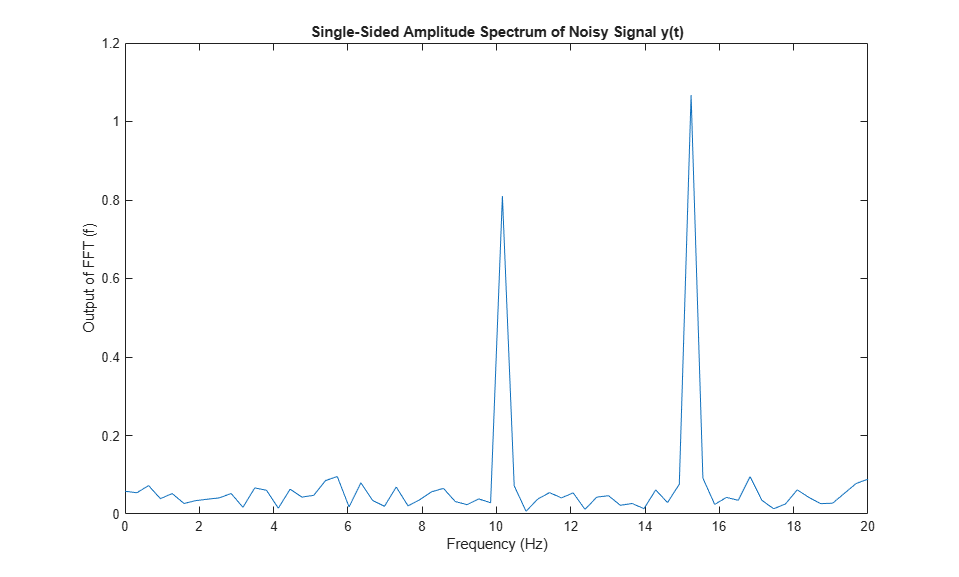Select frequencies that hold the majority of the energy in the signal. The `cumsum` function does not accept fixed-point arguments, so convert the data back to `double`.

```[Ysort,i] = sort(abs(double(transpose(Yf(1:N)))),1,'descend'); Ysort_d = double(Ysort); CumEnergy = sqrt(cumsum(Ysort_d.^2))/norm(Ysort_d); j = find(CumEnergy > 0.9, 1); disp(['Number of FFT coefficients that represent 90% of the ', ... 'total energy in the sequence: ', num2str(j)]) Yin = zeros(N,1); Yin(i(1:j)) = Yf(i(1:j)); ```
```Number of FFT coefficients that represent 90% of the total energy in the sequence: 4 ```

Write a function that creates and calls the IFFT System object™. You can generate HDL from this function.

```function [yOut,validOut] = HDLIFFT128(yIn,validIn) %HDLIFFT128 % Processes one sample of data using the dsphdl.IFFT System object(TM) % yIn is a fixed-point scalar or column vector. % validIn is a logical scalar. % You can generate HDL code from this function. persistent ifft128; if isempty(ifft128) ifft128 = dsphdl.IFFT('FFTLength',128); end [yOut,validOut] = ifft128(yIn,validIn); end ```

Compute the IFFT by calling the function for each data sample.

```Xt = zeros(1,3*N); validOut = false(1,3*N); for loop = 1:1:N [Xt(loop),validOut(loop)] = HDLIFFT128(complex(Yin(loop)),true); end for loop = N+1:1:3*N [Xt(loop),validOut(loop)] = HDLIFFT128(complex(0),false); end ```

Discard invalid output samples. Then inspect the output and compare it with the input signal. The original input is in green.

```Xt = Xt(validOut==1); Xt = bitrevorder(Xt); norm(x-transpose(Xt(1:N))) figure stem(real(Xt)) figure stem(real(x),'--g') ```
```ans = 0.7863 ```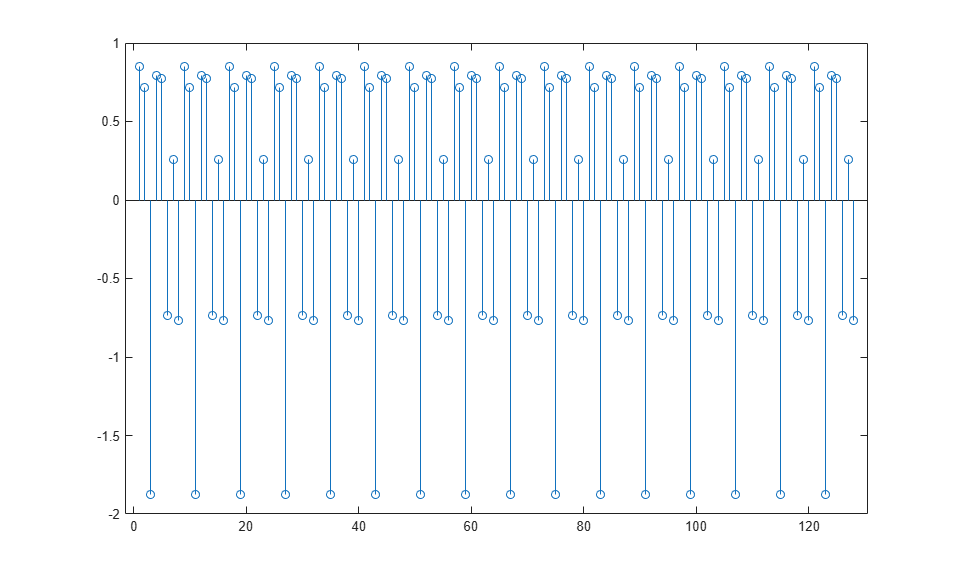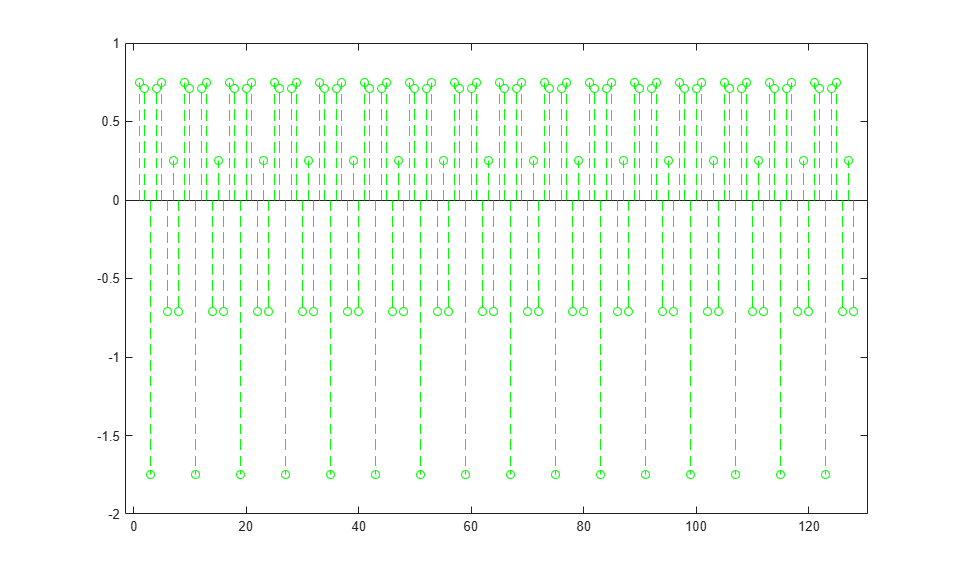Create the specifications and input signal. This example uses a 128-point FFT and computes the transform over 16 samples at a time.

```N = 128; V = 16; Fs = 40; t = (0:N-1)'/Fs; x = sin(2*pi*15*t) + 0.75*cos(2*pi*10*t); y = x + .25*randn(size(x)); y_fixed = sfi(y,32,24); y_vect = reshape(y_fixed,V,N/V); ```

Compute the FFT of the signal, to use as the input to the IFFT object.

```hdlfft = dsphdl.FFT('FFTLength',N); loopCount = getLatency(hdlfft,N,V)+N/V; Yf = zeros(V,loopCount); validOut = false(V,loopCount); for loop = 1:1:loopCount if ( mod(loop,N/V) == 0 ) i = N/V; else i = mod(loop,N/V); end [Yf(:,loop),validOut(loop)] = hdlfft(complex(y_vect(:,i)),(loop<=N/V)); end ```

Plot the single-sided amplitude spectrum.

```C = Yf(:,validOut==1); Yf_flat = C(:); Yr = bitrevorder(Yf_flat); plot(Fs/2*linspace(0,1,N/2),2*abs(Yr(1:N/2)/N)) title('Single-Sided Amplitude Spectrum of Noisy Signal y(t)') xlabel('Frequency (Hz)') ylabel('Output of FFT(f)') ```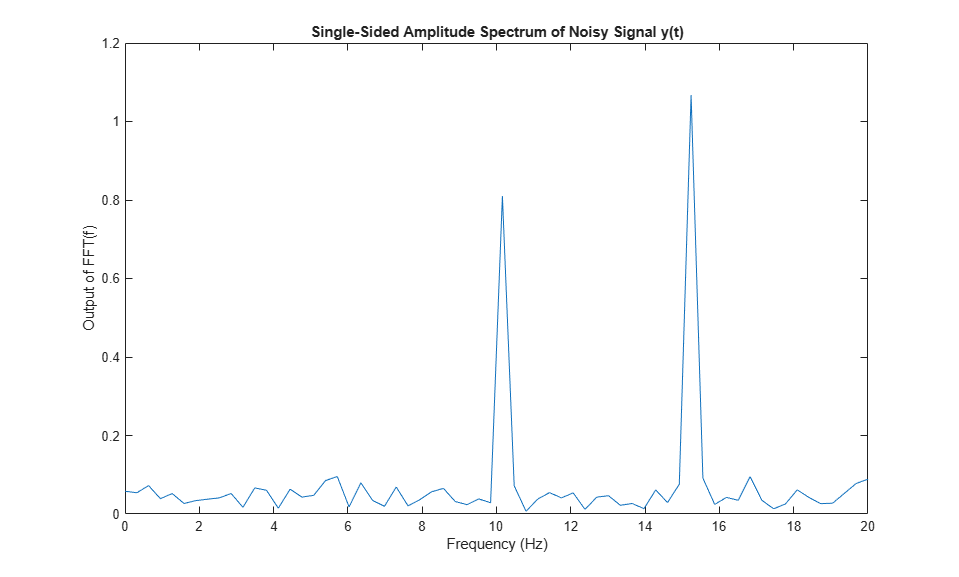Select frequencies that hold the majority of the energy in the signal. The `cumsum` function doesn't accept fixed-point arguments, so convert the data back to `double`.

```[Ysort,i] = sort(abs(double(Yr(1:N))),1,'descend'); CumEnergy = sqrt(cumsum(Ysort.^2))/norm(Ysort); j = find(CumEnergy > 0.9, 1); disp(['Number of FFT coefficients that represent 90% of the ', ... 'total energy in the sequence: ', num2str(j)]) Yin = zeros(N,1); Yin(i(1:j)) = Yr(i(1:j)); YinVect = reshape(Yin,V,N/V); ```
```Number of FFT coefficients that represent 90% of the total energy in the sequence: 4 ```

Write a function that creates and calls the IFFT System object™. You can generate HDL from this function.

```function [yOut,validOut] = HDLIFFT128V16(yIn,validIn) %HDLFFT128V16 % Processes 16-sample vectors of FFT data % yIn is a fixed-point column vector. % validIn is a logical scalar value. % You can generate HDL code from this function. persistent ifft128v16; if isempty(ifft128v16) ifft128v16 = dsphdl.IFFT('FFTLength',128) end [yOut,validOut] = ifft128v16(yIn,validIn); end ```

Compute the IFFT by calling the function for each data sample.

```Xt = zeros(V,loopCount); validOut = false(V,loopCount); for loop = 1:1:loopCount if ( mod(loop,N/V) == 0 ) i = N/V; else i = mod(loop,N/V); end [Xt(:,loop),validOut(loop)] = HDLIFFT128V16(complex(YinVect(:,i)),(loop<=N/V)); end ```
```ifft128v16 = dsphdl.IFFT with properties: FFTLength: 128 Architecture: 'Streaming Radix 2^2' ComplexMultiplication: 'Use 4 multipliers and 2 adders' BitReversedOutput: true BitReversedInput: false Normalize: true Use get to show all properties ```

Discard invalid output samples. Then inspect the output and compare it with the input signal. The original input is in green.

```C = Xt(:,validOut==1); Xt = C(:); Xt = bitrevorder(Xt); norm(x-Xt(1:N)) figure stem(real(Xt)) figure stem(real(x),'--g') ```
```ans = 0.7863 ```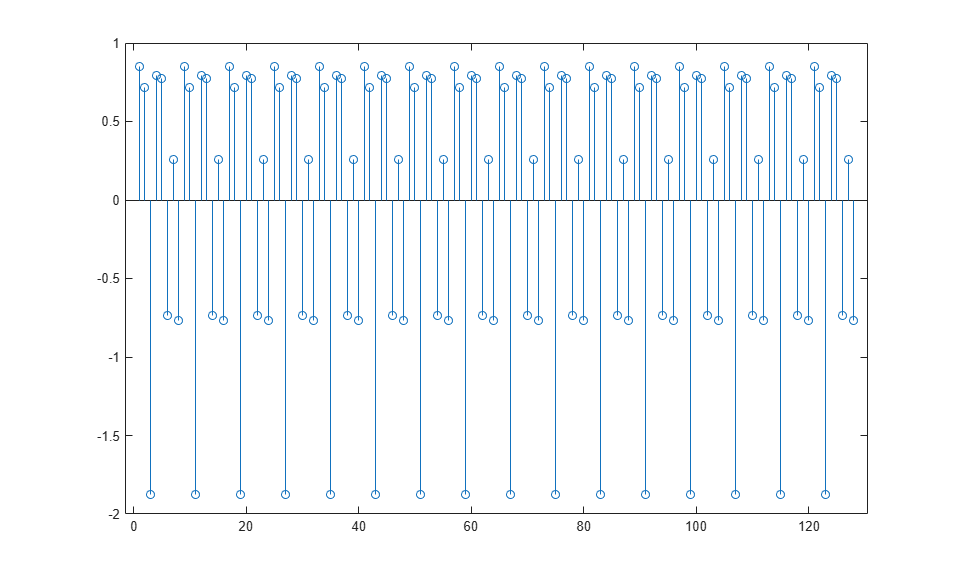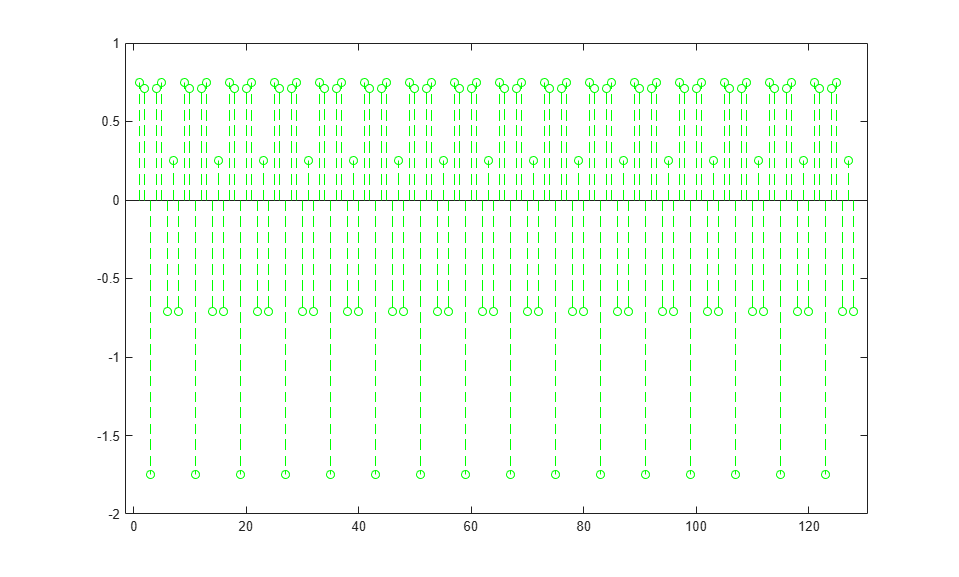The latency of the object varies with the FFT length and the vector size. Use the `getLatency` function to find the latency of a particular configuration. The latency is the number of cycles between the first valid input and the first valid output, assuming that the input is contiguous.

Create a new `dsphdl.IFFT` object and request the latency.

```hdlifft = dsphdl.IFFT('FFTLength',512); L512 = getLatency(hdlifft)```
```L512 = 599 ```

Request hypothetical latency information about a similar object with a different FFT length. The properties of the original object do not change. When you do not specify a vector length, the function assumes scalar input data.

`L256 = getLatency(hdlifft,256)`
```L256 = 329 ```
`N = hdlifft.FFTLength`
```N = 512 ```

Request hypothetical latency information of a similar object that accepts eight-sample vector input.

`L256v8 = getLatency(hdlifft,256,8)`
```L256v8 = 93 ```

Enable scaling at each stage of the IFFT. The latency does not change.

```hdlifft.Normalize = true; L512n = getLatency(hdlifft)```
```L512n = 599 ```

Request the same output order as the input order. This setting increases the latency because the object must collect the output before reordering.

```hdlifft.BitReversedOutput = false; L512r = getLatency(hdlifft)```
```L512r = 1078 ```

expand all

## Version History

Introduced in R2014b

expand all

Behavior changed in R2022a

Behavior changed in R2022a